# Curvature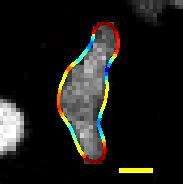A migrating wild-type Dictyostelium discoideum cell whose boundary is colored by curvature. Scale bar: 5µm;.

In mathematics, curvature is any of a number of loosely related concepts in different areas of geometry. Intuitively, curvature is the amount by which a geometric object such as a surface deviates from being a flat plane, or a curve from being straight as in the case of a line, but this is defined in different ways depending on the context. There is a key distinction between extrinsic curvature, which is defined for objects embedded in another space (usually a Euclidean space) – in a way that relates to the radius of curvature of circles that touch the object – and intrinsic curvature, which is defined in terms of the lengths of curves within a Riemannian manifold. This article deals primarily with the extrinsic concept.

The canonical example of extrinsic curvature is that of a circle, which has a curvature equal to the reciprocal of its radius everywhere. Smaller circles bend more sharply, and hence have higher curvature. The curvature of a smooth curve is defined as the curvature of its osculating circle at each point.

More commonly curvature is a scalar quantity, but one may also define a curvature vector that takes into account the direction of the bend as well as its sharpness. The curvature of more complex objects (such as surfaces or even curved n-dimensional spaces) is described by more complex objects from linear algebra, such as the general Riemann curvature tensor.

This article sketches the mathematical framework which describes the curvature of a curve embedded in a plane and the curvature of a surface in Euclidean space.

## Curvature of plane curves

Augustin-Louis Cauchy defined the centre of curvature C as the intersection point of two infinitely close normals to the curve, the radius of curvature as the distance from the point to C, and the curvature itself as the inverse of the radius of curvature.

Let C be a plane curve (the precise technical assumptions are given below). The curvature of C at a point is a measure of how sensitive its tangent line is to moving the point to other nearby points. There are a number of equivalent ways that this idea can be made precise.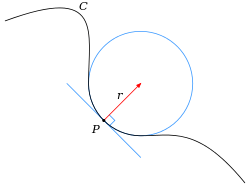One way is geometrical. It is natural to define the curvature of a straight line to be constantly zero. The curvature of a circle of radius R should be large if R is small and small if R is large. Thus the curvature of a circle is defined to be the reciprocal of the radius:Given any curve C and a point P on it, there is a unique circle or line which most closely approximates the curve near P, the osculating circle at P. The curvature of C at P is then defined to be the curvature of that circle or line. The radius of curvature is defined as the reciprocal of the curvature.

Another way to understand the curvature is physical. Suppose that a particle moves along the curve with unit speed. Taking the time s as the parameter for C, this provides a natural parametrization for the curve. The unit tangent vector T (which is also the velocity vector, since the particle is moving with unit speed) also depends on time. The curvature is then the magnitude of the rate of change of T. Symbolically,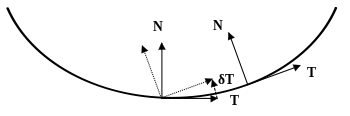The vectors T and N at two points on a plane curve, a translated version of the second frame (dotted), and the change in T: δT. δs is the distance between the points. In the limit dT/ds will be in the direction N and the curvature describes the speed of rotation of the frame.

This is the magnitude of the acceleration of the particle and the vector dT/ds is the acceleration vector. Geometrically, the curvature κ measures how fast the unit tangent vector to the curve rotates. If a curve keeps close to the same direction, the unit tangent vector changes very little and the curvature is small; where the curve undergoes a tight turn, the curvature is large.

These two approaches to the curvature are related geometrically by the following observation. In the first definition, the curvature of a circle is equal to the ratio of the angle of an arc to its length. Likewise, the curvature of a plane curve at any point is the limiting ratio of , an infinitesimal angle (in radians) between tangents to that curve at the ends of an infinitesimal segment of the curve, to the length of that segment ds, i.e., /ds. If the tangents at the ends of the segment are represented by unit vectors, it is easy to show that in this limit, the magnitude of the difference vector is equal to , which leads to the given expression in the second definition of curvature.

### Precise definition

Suppose that C is a twice continuously differentiable immersed plane curve, which here means that there exists a parametric representation of C by a pair of functions γ(t) = (x(t), y(t)) such that the first and second derivatives of x and y both exist and are continuous, andthroughout the domain. For such a plane curve, there exists a reparametrization with respect to arc length s. This is a parametrization of C such thatThe velocity vector T(s) is the unit tangent vector. The unit normal vector N(s), the curvature κ(s), the oriented or signed curvature k(s), and the radius of curvature R(s) are given byExpressions for calculating the curvature in arbitrary coordinate systems are given below.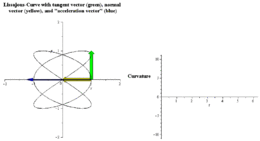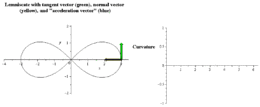Animations of the signed curvature and the acceleration vector T′(s)

### Signed curvature

The sign of the signed curvature k indicates the direction in which the unit tangent vector rotates as a function of the parameter along the curve. If the unit tangent rotates counterclockwise, then k > 0. If it rotates clockwise, then k < 0. So, for example, the sign of the curvature of a graph is the same as the sign of the second derivative (see below).

The signed curvature depends on the particular parametrization chosen for a curve. For example, the unit circle can be parametrised by (cos θ, sin θ) (counterclockwise, with k > 0), or by (cos(−θ), sin(−θ)) (clockwise, with k < 0). More precisely, the signed curvature depends only on the choice of orientation of an immersed curve. Every immersed curve in the plane admits two possible orientations.

### Local expressions

For a plane curve given parametrically in Cartesian coordinates as γ(t) = (x(t),y(t)), the curvature iswhere primes refer to derivatives ddt with respect to the parameter t. The signed curvature k isThe expression reflects the geometric meaning discussed above, that the curvature is influenced by the change amount of the tangent vector in the direction of the normal vector, asThese can be expressed in a coordinate-independent manner via### Curvature of a graph

For the less general case of a plane curve given explicitly as y = f(x), and now using primes for derivatives ddx with respect to coordinate x, the curvature isand the signed curvature isThis quantity is common in physics and engineering; for example, in the equations of bending in beams, the one-dimensional vibration of a tense string, approximations to the fluid flow around surfaces (in aeronautics), and the free surface boundary conditions in ocean waves. In such applications, the assumption is almost always made that the slope is small compared with unity, so that the approximation:may be used. This approximation yields a straightforward linear equation describing the phenomenon.

If a curve is defined in polar coordinates as r(θ), then its curvature iswhere here the prime refers to differentiation with respect to θ.

### Examples

Consider the parabola y = x2. We can parametrize the curve simply as γ(t) = (t,t2) = (x,y). If we use primes for derivatives with respect to the parameter t, thenSubstituting and dropping unnecessary absolute values, getAnd the same result may be obtained immediately from the above formula of the curvature of a graph, without parametrizing.

A Lissajous curve with a 3:2 ratio of frequencies can be parametrized in this way:Applying the formula it turns out to have signed curvature:(For more details on this example see osculating circle.)

## Curvature of space curves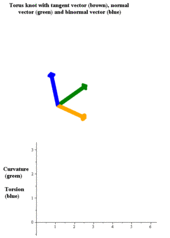Animation of the curvature and the acceleration vector T′(s)

As in the case of curves in two dimensions, the curvature of a regular space curve C in three dimensions (and higher) is the magnitude of the acceleration of a particle moving with unit speed along a curve. Thus if γ(s) is the arc-length parametrization of C then the unit tangent vector T(s) is given byand the curvature is the magnitude of the acceleration:The direction of the acceleration is the unit normal vector N(s), which is defined byThe plane containing the two vectors T(s) and N(s) is called the osculating plane to the curve at γ(s). The curvature has the following geometrical interpretation. There exists a circle in the osculating plane tangent to γ(s) whose Taylor series to second order at the point of contact agrees with that of γ(s). This is the osculating circle to the curve. The radius of the circle R(s) is called the radius of curvature, and the curvature is the reciprocal of the radius of curvature:The tangent, curvature, and normal vector together describe the second-order behavior of a curve near a point. In three-dimensions, the third order behavior of a curve is described by a related notion of torsion, which measures the extent to which a curve tends to move in a helical path in space. The torsion and curvature are related by the Frenet–Serret formulas (in three dimensions) and their generalization (in higher dimensions).

### Local expressions

For a parametrically-defined space curve in three dimensions given in Cartesian coordinates by γ(t) = (x(t), y(t), z(t)), the curvature iswhere the prime denotes differentiation with respect to the parameter t. This can be expressed independently of the coordinate system by means of the formulawhere × is the vector cross product. Equivalently,Here the T denotes the matrix transpose. This last formula is also valid for the curvature of curves in a Euclidean space of any dimension.

### Curvature from arc and chord length

Given two points P and Q on C, let s(P,Q) be the arc length of the portion of the curve between P and Q and let d(P,Q) denote the length of the line segment from P to Q. The curvature of C at P is given by the limitwhere the limit is taken as the point Q approaches P on C. The denominator can equally well be taken to be d(P,Q)3. The formula is valid in any dimension. Furthermore, by considering the limit independently on either side of P, this definition of the curvature can sometimes accommodate a singularity at P. The formula follows by verifying it for the osculating circle.

## Curvature of curves on surfaces

When a one-dimensional curve lies on a two dimensional surface embedded in three dimensions 3, further measures of curvature are available, which take the surface's unit normal vector, u into account. These are the normal curvature, geodesic curvature and geodesic torsion. Any non-singular curve on a smooth surface will have its tangent vector T lying in the tangent plane of the surface orthogonal to the normal vector. The normal curvature, kn, is the curvature of the curve projected onto the plane containing the curve's tangent T and the surface normal u; the geodesic curvature, kg, is the curvature of the curve projected onto the surface's tangent plane; and the geodesic torsion (or relative torsion), τr, measures the rate of change of the surface normal around the curve's tangent.

Let the curve be a unit speed curve and let t = u × T so that T, u, t form an orthonormal basis: the Darboux frame. The above quantities are related by:### Principal curvature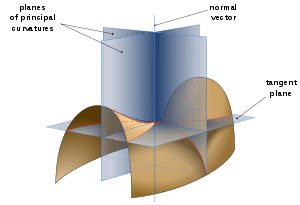Saddle surface with normal planes in directions of principal curvatures
Main article: Principal curvature

All curves with the same tangent vector will have the same normal curvature, which is the same as the curvature of the curve obtained by intersecting the surface with the plane containing T and u. Taking all possible tangent vectors, the maximum and minimum values of the normal curvature at a point are called the principal curvatures, k1 and k2, and the directions of the corresponding tangent vectors are called principal normal directions.

## Curvature of surfaces

For a broader coverage related to this topic, see Differential geometry of surfaces.

### Normal sections

Curvature can be evaluated along surface normal sections, similar to #Curves on surfaces above (see, e.g., Earth radius of curvature).

### Gaussian curvature

Main article: Gaussian curvature

In contrast to curves, which do not have intrinsic curvature, but do have extrinsic curvature (they only have a curvature given an embedding), surfaces can have intrinsic curvature, independent of an embedding. The Gaussian curvature, named after Carl Friedrich Gauss, is equal to the product of the principal curvatures, k1k2. It has a dimension of length−2 and is positive for spheres, negative for one-sheet hyperboloids and zero for planes. It determines whether a surface is locally convex (when it is positive) or locally saddle-shaped (when it is negative).

Gaussian curvature is an intrinsic property of the surface, meaning it does not depend on the particular embedding of the surface; intuitively, this means that ants living on the surface could determine the Gaussian curvature. For example, an ant living on a sphere could measure the sum of the interior angles of a triangle and determine that it was greater than 180 degrees, implying that the space it inhabited had positive curvature. On the other hand, an ant living on a cylinder would not detect any such departure from Euclidean geometry; in particular the ant could not detect that the two surfaces have different mean curvatures (see below), which is a purely extrinsic type of curvature.

Formally, Gaussian curvature only depends on the Riemannian metric of the surface. This is Gauss's celebrated Theorema Egregium, which he found while concerned with geographic surveys and mapmaking.

An intrinsic definition of the Gaussian curvature at a point P is the following: imagine an ant which is tied to P with a short thread of length r. It runs around P while the thread is completely stretched and measures the length C(r) of one complete trip around P. If the surface were flat, she would find C(r) = 2πr. On curved surfaces, the formula for C(r) will be different, and the Gaussian curvature K at the point P can be computed by the Bertrand–Diquet–Puiseux theorem asThe integral of the Gaussian curvature over the whole surface is closely related to the surface's Euler characteristic; see the Gauss–Bonnet theorem.

The discrete analog of curvature, corresponding to curvature being concentrated at a point and particularly useful for polyhedra, is the (angular) defect; the analog for the Gauss–Bonnet theorem is Descartes' theorem on total angular defect.

Because (Gaussian) curvature can be defined without reference to an embedding space, it is not necessary that a surface be embedded in a higher-dimensional space in order to be curved. Such an intrinsically curved two-dimensional surface is a simple example of a Riemannian manifold.

### Mean curvature

Main article: Mean curvature

The mean curvature is an extrinsic measure of curvature equal to half the sum of the principal curvatures, k1 + k2/2. It has a dimension of length−1. Mean curvature is closely related to the first variation of surface area, in particular a minimal surface such as a soap film, has mean curvature zero and a soap bubble has constant mean curvature. Unlike Gauss curvature, the mean curvature is extrinsic and depends on the embedding, for instance, a cylinder and a plane are locally isometric but the mean curvature of a plane is zero while that of a cylinder is nonzero.

### Second fundamental form

The intrinsic and extrinsic curvature of a surface can be combined in the second fundamental form. This is a quadratic form in the tangent plane to the surface at a point whose value at a particular tangent vector X to the surface is the normal component of the acceleration of a curve along the surface tangent to X; that is, it is the normal curvature to a curve tangent to X (see above). Symbolically,where N is the unit normal to the surface. For unit tangent vectors X, the second fundamental form assumes the maximum value k1 and minimum value k2, which occur in the principal directions u1 and u2, respectively. Thus, by the principal axis theorem, the second fundamental form isThus the second fundamental form encodes both the intrinsic and extrinsic curvatures.

### Shape operator

Further information: Shape operator

A related notion of curvature is the shape operator, which is a linear operator from the tangent plane to itself. When applied to a tangent vector X to the surface, the shape operator is the tangential component of the rate of change of the normal vector when moved along a curve on the surface tangent to X. The principal curvatures are the eigenvalues of the shape operator, and in fact the shape operator and second fundamental form have the same matrix representation with respect to a pair of orthonormal vectors of the tangent plane. The Gauss curvature is thus the determinant of the shape tensor and the mean curvature is half its trace.

## Curvature of space

"Curvature of space" redirects here. It is not to be confused with Curvature of space-time.

By extension of the former argument, a space of three or more dimensions can be intrinsically curved. The curvature is intrinsic in the sense that it is a property defined at every point in the space, rather than a property defined with respect to a larger space that contains it. In general, a curved space may or may not be conceived as being embedded in a higher-dimensional ambient space; if not then its curvature can only be defined intrinsically.

After the discovery of the intrinsic definition of curvature, which is closely connected with non-Euclidean geometry, many mathematicians and scientists questioned whether ordinary physical space might be curved, although the success of Euclidean geometry up to that time meant that the radius of curvature must be astronomically large. In the theory of general relativity, which describes gravity and cosmology, the idea is slightly generalised to the "curvature of spacetime"; in relativity theory spacetime is a pseudo-Riemannian manifold. Once a time coordinate is defined, the three-dimensional space corresponding to a particular time is generally a curved Riemannian manifold; but since the time coordinate choice is largely arbitrary, it is the underlying spacetime curvature that is physically significant.

Although an arbitrarily curved space is very complex to describe, the curvature of a space which is locally isotropic and homogeneous is described by a single Gaussian curvature, as for a surface; mathematically these are strong conditions, but they correspond to reasonable physical assumptions (all points and all directions are indistinguishable). A positive curvature corresponds to the inverse square radius of curvature; an example is a sphere or hypersphere. An example of negatively curved space is hyperbolic geometry. A space or space-time with zero curvature is called flat. For example, Euclidean space is an example of a flat space, and Minkowski space is an example of a flat spacetime. There are other examples of flat geometries in both settings, though. A torus or a cylinder can both be given flat metrics, but differ in their topology. Other topologies are also possible for curved space. See also shape of the universe.

## Generalizations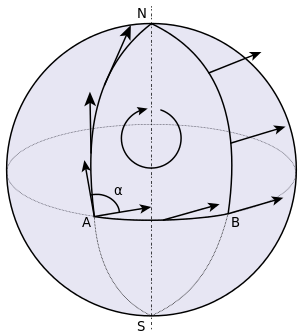Parallel transporting a vector from ANBA yields a different vector. This failure to return to the initial vector is measured by the holonomy of the surface.

The mathematical notion of curvature is also defined in much more general contexts. Many of these generalizations emphasize different aspects of the curvature as it is understood in lower dimensions.

One such generalization is kinematic. The curvature of a curve can naturally be considered as a kinematic quantity, representing the force felt by a certain observer moving along the curve; analogously, curvature in higher dimensions can be regarded as a kind of tidal force (this is one way of thinking of the sectional curvature). This generalization of curvature depends on how nearby test particles diverge or converge when they are allowed to move freely in the space; see Jacobi field.

Another broad generalization of curvature comes from the study of parallel transport on a surface. For instance, if a vector is moved around a loop on the surface of a sphere keeping parallel throughout the motion, then the final position of the vector may not be the same as the initial position of the vector. This phenomenon is known as holonomy. Various generalizations capture in an abstract form this idea of curvature as a measure of holonomy; see curvature form. A closely related notion of curvature comes from gauge theory in physics, where the curvature represents a field and a vector potential for the field is a quantity that is in general path-dependent: it may change if an observer moves around a loop.

Two more generalizations of curvature are the scalar curvature and Ricci curvature. In a curved surface such as the sphere, the area of a disc on the surface differs from the area of a disc of the same radius in flat space. This difference (in a suitable limit) is measured by the scalar curvature. The difference in area of a sector of the disc is measured by the Ricci curvature. Each of the scalar curvature and Ricci curvature are defined in analogous ways in three and higher dimensions. They are particularly important in relativity theory, where they both appear on the side of Einstein's field equations that represents the geometry of spacetime (the other side of which represents the presence of matter and energy). These generalizations of curvature underlie, for instance, the notion that curvature can be a property of a measure; see curvature of a measure.

Another generalization of curvature relies on the ability to compare a curved space with another space that has constant curvature. Often this is done with triangles in the spaces. The notion of a triangle makes senses in metric spaces, and this gives rise to CAT(k) spaces.

## Notes

1. Kline, Morris. Calculus: An Intuitive and Physical Approach (2nd ed.). p. 458.
2. Pressley, Andrew. Elementary Differential Geometry (1st ed.). p. 29.
3. Pogorelov, A. V. Differential Geometry (1st ed.). p. 49.
4. Kennedy, John (2011). "The Arc Length Parametrization of a Curve".
5. Kobayashi, S.; Nomizu, K. Foundations of Differential Geometry. Wiley Interscience. vol. 1 ch. 2–3.
6. Henderson; Taimina. Experiencing Geometry (3rd ed.). p. 98–99.

## ReferencesWikimedia Commons has media related to Graphical illustrations of the curvature of curves.Look up curvature in Wiktionary, the free dictionary.